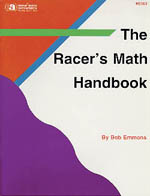The Racer's Math HandbookBy: Bob Emmons
Many of the racer's math formulas compiled into an easily understood guide. Explains: Use of basic formulas, rules of algebra, basic geometry, transmission and rear end math, engine formulas -- horsepower, compression ratio, carburetor sizing, etc., chassis and handling formulas -- brake sizing, lateral acceleration, weight distribution & weight transfer, spring rates of coils, torsion bars, sway bars, CGH, etc. Also included is an easy-to-use computer program (Windows format) that has every formula in the book & more.
\$29.95
Product Number: S193C
Media: Book